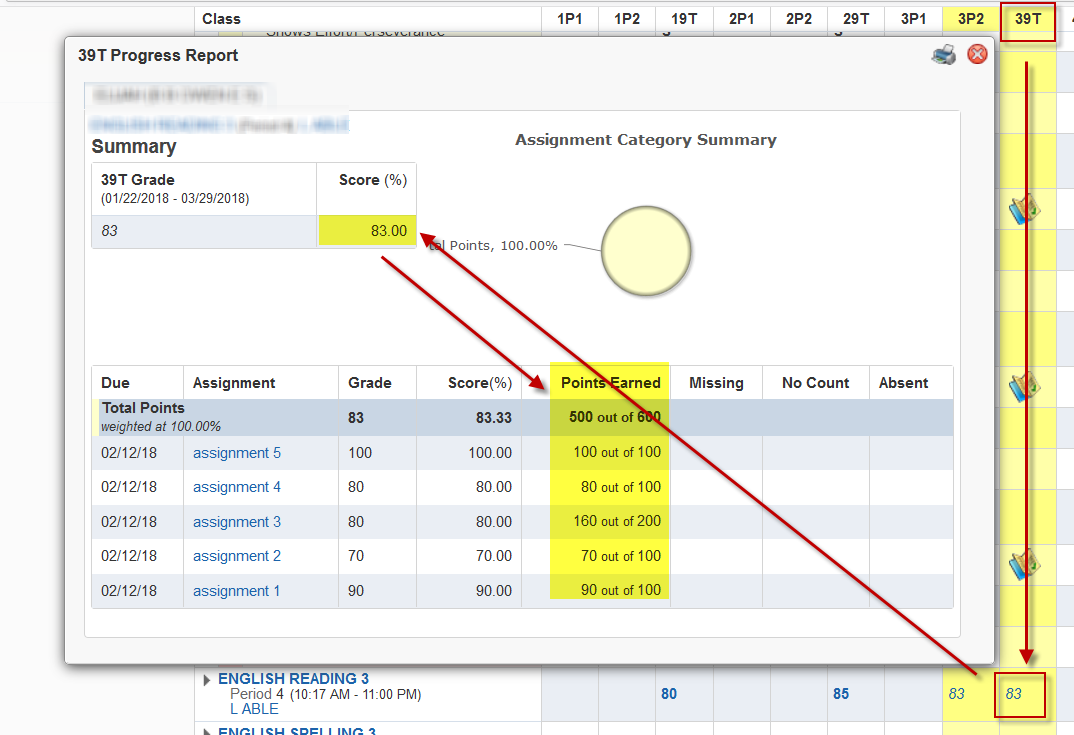### Grade Calculations in Skyward (Elementary)Submitted 2/12/2018 by LISD Skyward Support You need to log in to be able to download files

The grade calculations in Skyward are a combination of district default calculation settings and the setup in an individual teacher's gradebook. The district default calcuation settings are described below. For specific questions regarding grading in a teacher's class, you will need to contact the teacher through phone or by email using the links in Family Access for Message Center or direct email to the teacher.

## ELEMENTARY

9 WEEK GRADES (there are a total of 4)

Each nine weeks is calculated based on the calculation of the total points earned divided by the total points possible for all assignments in the term. Progress report grades are snapshots of the grade at a point in time and are NOT USED in calculating the 9 week grade.

EXAMPLE: The class has 5 assignments each with a maximum score of 100 points. A teacher can weight an individual assignment using a multiplier so points can be doubled for that assignment:

Assignment 1  Points possible= 100     student received a 90

Assignment 2  Points possible= 100     student received a 70

Assignment 3  Points possible= 100 (weighted "2" = 2 x100 for 200 possible points)     student received an 80 (weighted "2" = 2 x 80 for 160 earned points)

Assignment 4  Points possible= 100     student received a 80

Assignment 5  Points possible= 100     student received a 100

Calculation: points earned = 500  Points possible = 600 the average for this student would be: .83 (rounded to 2) x 100 for a 9 week average of 83

END OF YEAR GRADE (EOY) (does not appear until the start of the 4th 9 weeks)

Once a grade is posted for a 9 weeks, it becomes the official grade and will remain unchanged unless a teacher requests the change through a process in Skyward. Changes in gradebooks do not change the official grade used in the calculations until they do so.

EXAMPLE: The student received the following grades (if they drop a class in the year, the teachers or the new class MUST bring in those 9 weeks grads in order for the calculation to include them)

1st 9 week= 80

2nd 9 week = 90

3rd 9 week = 100

4th 9 week = 100

Calculation: 80 + 90 + 100 + 100= 370 which is divided by 4 = 92.5, rounded up for and end of year grade of 93.